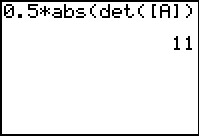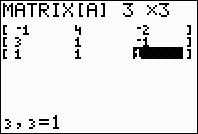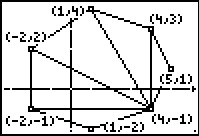••• ##### Device
• TI-83 Plus Family
• TI-84 Plus
• TI-84 Plus Silver Edition
•TI-84 Plus C Silver Edition
•TI-84 Plus CE
• ##### Software

TI Connect™
TI Connect™ CE

• ##### Report an Issue

Algebra II: Determining Area
by Texas Instruments#### Overview

Students use the area formula involving the determinant of a matrix.

#### Key Steps

•Students will use the area formula involving the determinant of a matrix. For the area of a triangle, they will compare their answer found using the formula to the one found using the Area tool in the Cabri™ Jr. application.

•Next, students will investigate the area of a convex polygon. They will divide the heptagon into triangles and then find the area of each triangle using the formula from the first problem. By summing all the areas of the triangles, students will have calculated the area of the heptagon.

•After using Cabri Jr. and triangle area formula to find the area of quadrilateral given the coordinates of its vertices, students will find a formula, using a 4 by 4 matrix, for the area of any quadrilateral through trial and error.